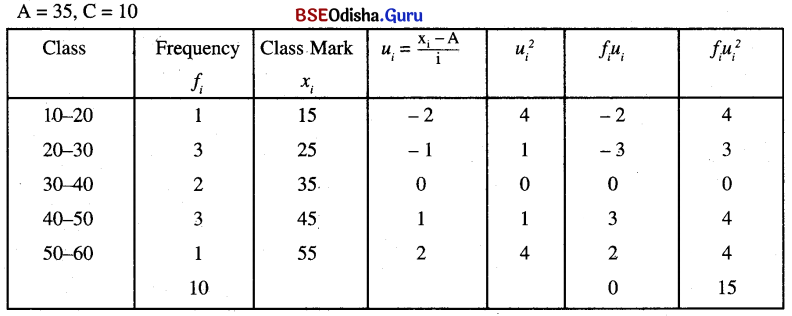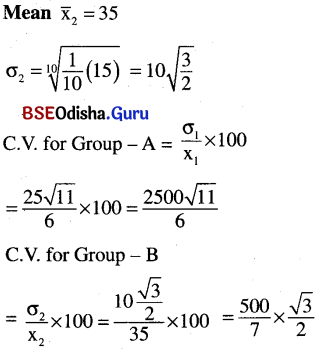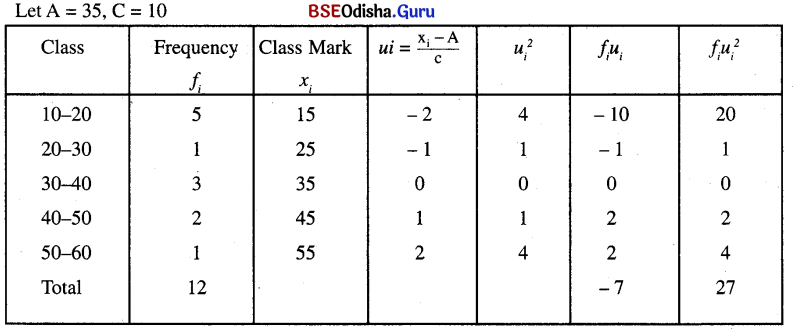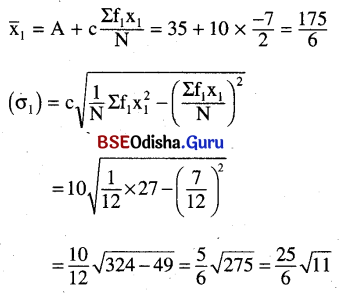# CHSE Odisha Class 11 Math Notes Chapter 15 Statistics

Odisha State Board CHSE Odisha Class 11 Math Notes Chapter 15 Statistics will enable students to study smartly.

## CHSE Odisha 11th Class Math Notes Chapter 15 Statistics

Measures Of Central Tendency:
A measure of central tendency or average is a value, that is the representative of whole data and signifies its characteristics.
Different measures of central tendency are: (a) Mean (b) Median (c) Mode.

(a) Mean (Arithmetic Mean):
Mean of ungrouped data: The mean of ‘n‘ observations x1, x2 …..xn = $$\bar{x} \frac{\sum_{i=1}^n x_i}{N}$$

Mean of grouped data:
(i) Direct Method
If xi are the mid values of the intervals with frequency fi then the mean $$\bar{x}=\frac{1}{N} \sum_{i=1}^n f_i x_i$$

(ii) Shortcut Methods:
(1) Assumed mean method
Mean = $$\overline{\mathrm{x}}=\mathrm{A}+\frac{1}{\mathrm{~N}} \sum_{\mathrm{i}=1}^{\mathrm{n}} \mathrm{f}_{\mathrm{i}} \mathrm{d}_{\mathrm{i}}$$
where A = the assumed mean ⇒ di = xi – A

(iii) Step Deviation Method:
Mean = $$\overline{\mathrm{x}}=\mathrm{A}+\frac{\mathrm{C}}{\mathrm{N}} \sum_{\mathrm{i}=1}^{\mathrm{n}} \mathrm{f}_{\mathrm{i}} \mathrm{u}_{\mathrm{i}}$$
where A = The assumed mean, C = Class width
ui = $$\frac{d_i}{C}=\frac{x_i-A}{C}$$

(b) Median
(i) Median of ungrouped data:
Let n is the number of observation.
Arrange the observations in ascending or descending order.
⇒ If n is odd, Median = $$\left(\frac{\mathrm{n}+1}{2}\right)^{\mathrm{th}}$$ observation.
⇒ If n is even, Median = $$\frac{\left(\frac{\mathrm{n}+1}{2}\right)^{\mathrm{th}} \text { observation }+\left(\frac{\mathrm{n}}{2}+1\right)^{\mathrm{th}} \text { observation }}{2}$$

(ii) Median of grouped data

• Get $$\frac{\mathrm{N}}{2}$$ and cummulative frequencies of all classes.
• Get the Median class.
Median class = The class whose cumulative frequency is just greater than (or near to) $$\frac{\mathrm{N}}{2}$$.
Median = l + $$\frac{\mathrm{m}-\mathrm{c}}{\mathrm{fm}}$$ × h,
where l = lower limit of median class .
h = Class width of median class M = $$\frac{\mathrm{N}}{2}$$.
c = Cummulative frequency of the class preceeding the median class.
fm = Frequency of the median class.

(c) Mode
Mode is the most frequent value.
⇒ We can find mode using the empirical formula:
Mode = 3 Median – 2 Mean.
(i) Mode for Grouped data
⇒ Get the Modal class: It is the class with maximum frequency.
Mode = $$l+\frac{\mathrm{f}_{\mathrm{m}}-\mathrm{f}_1}{2 \mathrm{f}_{\mathrm{m}}-\mathrm{f}_1-\mathrm{f}_2} \times \mathrm{c}$$
where l = lower limit of modal class.
fm = Frequency of modal class.
f1 = Frequency of the class just preceeding modal class.
f2 = Frequency of the class just suceeding modal class.Measure Of Dispersion:
The variability or scatter or spreading of data is known as dispersion.

Some of the measures of dispersion are:

(a) Range
(b) Mean deviation
(c) Variance
(d) Standard deviation

(a) Mean deviation: Mean deviation is the mean of absolute deviations of all observations from a central value (Mean or Median).

For Group – B
A = 35, C = 10As C. V of Group – A is more, the data for group – A is more dispersed

For Group – AAnalysis Of Frequency Distribution:
Coefficient of variation (C. V) = $$\frac{\sigma}{x}$$ × 100

Note:

• The distribution with greater C. V is more variable or dispersed and lesser C. V is less variable or more consistent.
• If two distributions have same mean then they can be compared on the basis of their standard deviation.